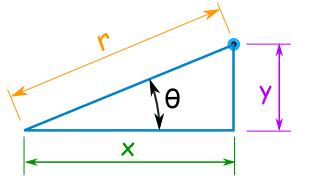# Conversion from polar coordinate to Cartesian coordinate

We know about Cartesian(or Rectangular, or x-y) coordinate system. However, as we will see, this is not always the easiest coordinate system to work in.
Coordinate systems are really nothing more than a way to define a point in space. For instance in the Cartesian coordinate system at point is given the coordinates (x,y) and we use this to define the point by starting at the origin and then moving x units horizontally followed by y units vertically.

This is not, however, the only way to define a point in two dimensional space. Instead of moving vertically and horizontally from the origin to get to the point we could instead go straight out of the origin until we hit the point and then determine the angle this line makes with the positive x-axis. We could then use the distance of the point from the origin and the amount we needed to rotate from the positive x-axis as the coordinates of the point.
Coordinates in this form are called polar coordinates.# Conversion from polar coordinate to Cartesian coordinate

There are some formulas to convert it``````So to convert from Cartesian Coordinates (x,y) to Polar Coordinates (r,θ):

r = √ ( x2 + y2 )
θ = tan-1 ( y / x )

and to convert from Polar Coordinates (r,θ) to Cartesian Coordinates (x,y) :
x = r × cos( θ )
y = r × sin( θ )``````

Now it’s time to code

```#include<stdio.h>
#include<conio.h>
#include<iostream.h>
#include<math.h>
#define PI 3.1415926
class polar;
class cart
{
float x,y;
public:
friend class polar;
cart()
{
x=0;y=0;
}
cart(float q,float w)
{
x=q;
y=w;
}
cart(polar p);
int print()
{
cout<<"value of"<<endl<<"x="<<x<<endl<<"y="<<y;
}
};
class polar
{
float r,theta;
public:
friend class cart;
polar()
{
r=0;theta=0;
}
polar(float e,float r)
{
r=e;
theta=r*PI/180;
}
polar(cart c)
{
r=sqrt(c.x*c.x+c.y*c.y);
theta=atan(c.y/c.x);
}
int print()
{
cout<<"value of"<<endl<<"r="<<r<<endl<<"theta="<<theta*180/PI;
}
};
cart::cart(polar p)
{
x=p.r*cos(p.theta);
y=p.r*sin(p.theta);
}
int main()
{
cart c(1,1),c1;
polar p(2,30),p1;
c1=p;
c1.print();
p1=c;
p1.print();

}```
```#include<iostream.h>
#include<math.h>
#define PI 3.14
using namespace std;
class cartesian
{
public:
float x,y;
cartesian()
{
x=3,y=3;
}
void print()
{
cout<<"value of new x="<<x<<"\n";
cout<<"value of new y="<<y<<"\n";
}
};
class polar
{
public:
float r,theta;

polar()
{
r=2,theta=30*PI/180;
}
operator cartesian()
{
cartesian A;
A.x=r*cos(theta);
A.y=r*sin(theta);
return A;
}
polar( cartesian A)
{
r=sqrt(A.x*A.x+A.y*A.y);
theta=atan(A.y/A.x);
}
void print()
{
cout<<"value of new r="<<r<<"\n";
cout<<"value of new theta="<<theta*180/PI;
}
};
int main()
{
cartesian a,b;
polar p1,p2;
b=p1;
b.print();
p2=a;
p2.print();
}
```
```#include<stdio.h>
#include<iostream.h>
#include<math.h>
#define PI 3.1415926
using namespace std;
class polar;
class cartesian
{
public:
float x,y;
cartesian()
{
x=0,y=0;
}
/*operator polar()
{
polar p;
p.r=sqrt(x*x+y*y);
p.theta=atan(y/x);
return p;
}*/
cartesian(polar p);

void print()
{
cout<<"value of new x="<<x<<"\n";
cout<<"value of new y="<<y<<"\n";
}
};

class polar
{
public:
float r,theta;

polar(float a,float b)
{
r=a,theta=b*PI/180;
}
polar( cartesian c)
{
r=sqrt(c.x*c.x+c.y*c.y);
theta=atan(c.y/c.x);
)
void print()
{
cout<<"value of new r="<<r<<"\n";
cout<<"value of new theta="<<theta*180/PI;
}
};
cartesian::cartesian(polar p)
{
x=p.r*cos(p.theta);
y=p.r*sin(p.theta);
}
int main()
{
cartesian A;
polar p;
A=p;
A.print();
p=A;
p.print();
}
```All rights reserved. No part of this Post may be copied, distributed, or transmitted in any form or by any means, without the prior written permission of the website admin, except in the case of brief quotations embodied in critical reviews and certain other noncommercial uses permitted by copyright law. For permission requests, write to the owner, addressed “Attention: Permissions Coordinator,” to the admin @coderinme

### hasectic

A web developer(Front end and Back end), and DBA at csdamu.com. Currently working as Salesforce Developer @ Tech Matrix IT Consulting Private Limited. Check me @about.me/s.saifi